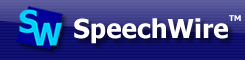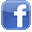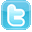Home     LIVE!     Features     Order     Calendar     Results     Your account     Manager loginTeam results

Milby High School Buffalo
A High School team from Texas

Viewing results from 2017-2018. Change season:

Click on a tournament name to view results from that tournament, a competitor name to view results for that competitor, an event to view the team's results in that event, or a final place to view the other results in that event at that tournament.

 Team sweepstakes results 6 HISD 2k17 UIL HS Meet 1 0 11 HISD 2k17 UIL HS Meet 2 0 12 HISD 2k17 UIL HS Meet 5 0 16 HISD 2k17 UIL HS Meet 3 0 16 HISD 2k17 UIL HS Meet 4 0

Competitor results

 Competitor Place Event Division HISD 2k17 UIL HS Meet 2 - Final results Erik Rodriguez Fin. Number Sense (NS) Open Thanh Do Fin. Calculator Applications (CALC) Open Erik Rodriguez Fin. Mathematics (MATH) Open Thanh Do Fin. Mathematics (MATH) Open Erik Rodriguez Fin. Science (SCI) Open Tien Vo Fin. Number Sense (NS) Open Dat Do Fin. Number Sense (NS) Open Erik Rodriguez Fin. Calculator Applications (CALC) Open Thanh Do Fin. Number Sense (NS) Open Dat Do Fin. Mathematics (MATH) Open Phuong Nguyen Fin. Science (SCI) Open Phuong Nguyen Fin. Calculator Applications (CALC) Open Dat Do Fin. Science (SCI) Open Dat Do Fin. Calculator Applications (CALC) Open Phuong Nguyen Fin. Number Sense (NS) Open Tien Vo Fin. Mathematics (MATH) Open Tien Vo Fin. Calculator Applications (CALC) Open Phuong Nguyen Fin. Mathematics (MATH) Open Tien Vo Fin. Science (SCI) Open Thanh Do Fin. Science (SCI) Open HISD 2k17 UIL HS Meet 4 - Final results Rogelio Mosqueda Fin. Number Sense (NS) Open Erik Rodriguez Fin. Mathematics (MATH) Open Erik Rodriguez Fin. Number Sense (NS) Open Thanh Do Fin. Calculator Applications (CALC) Open Thanh Do Fin. Mathematics (MATH) Open Tien Vo Fin. Number Sense (NS) Open Elijah Lozano Fin. Number Sense (NS) Open Jesus Garcia Fin. Number Sense (NS) Open Erik Rodriguez Fin. Calculator Applications (CALC) Open Tien Vo Fin. Calculator Applications (CALC) Open Rogelio Mosqueda Fin. Science (SCI) Open Rogelio Mosqueda Fin. Calculator Applications (CALC) Open Rogelio Mosqueda Fin. Mathematics (MATH) Open Thanh Do Fin. Number Sense (NS) Open Jacinda Servantes Fin. Number Sense (NS) Open Jesus Garcia Fin. Calculator Applications (CALC) Open Jacinda Servantes Fin. Science (SCI) Open Jacinda Servantes Fin. Calculator Applications (CALC) Open Elijah Lozano Fin. Calculator Applications (CALC) Open Tien Vo Fin. Mathematics (MATH) Open Elijah Lozano Fin. Science (SCI) Open Jesus Garcia Fin. Science (SCI) Open Erik Rodriguez Fin. Science (SCI) Open Elijah Lozano Fin. Mathematics (MATH) Open Tien Vo Fin. Science (SCI) Open Jesus Garcia Fin. Mathematics (MATH) Open Thanh Do Fin. Science (SCI) Open Jacinda Servantes Fin. Mathematics (MATH) Open HISD 2k17 UIL HS Meet 5 - Final results Erik Rodriguez Fin. Number Sense (NS) Open Rogelio Mosqueda Fin. Number Sense (NS) Open Erik Rodriguez Fin. Calculator Applications (CALC) Open Dat Do Fin. Number Sense (NS) Open Erik Rodriguez Fin. Mathematics (MATH) Open Jesus Garcia Fin. Number Sense (NS) Open Thanh Do Fin. Calculator Applications (CALC) Open Erik Rodriguez Fin. Science (SCI) Open Tien Vo Fin. Number Sense (NS) Open Rogelio Mosqueda Fin. Mathematics (MATH) Open Tien Vo Fin. Calculator Applications (CALC) Open Jacinda Servantes Fin. Number Sense (NS) Open Rogelio Mosqueda Fin. Calculator Applications (CALC) Open Thanh Do Fin. Mathematics (MATH) Open Thanh Do Fin. Number Sense (NS) Open Jacinda Servantes Fin. Science (SCI) Open Jesus Garcia Fin. Calculator Applications (CALC) Open Jacinda Servantes Fin. Calculator Applications (CALC) Open Rogelio Mosqueda Fin. Science (SCI) Open Jesus Garcia Fin. Science (SCI) Open Dat Do Fin. Calculator Applications (CALC) Open Jesus Garcia Fin. Mathematics (MATH) Open Tien Vo Fin. Mathematics (MATH) Open Jacinda Servantes Fin. Mathematics (MATH) Open Thanh Do Fin. Science (SCI) Open Dat Do Fin. Mathematics (MATH) Open Tien Vo Fin. Science (SCI) Open Dat Do Fin. Science (SCI) Open